## Quantifying the cost of simultaneous non-parametric approximation of several samples

Given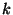samples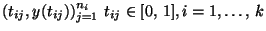we consider the problem of finding a function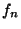, if any, which is simultaneously an adequate approximation for allsamples. Possible measures of cost are the number of local extremes, the total variation ofand its derivatives and the supremum norm of derivatives of. The costs of a joint approximation can be comparedwith the costs of approximating each sample separately. The concept of approximation used is based on the means of residuals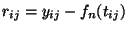over a family of subsets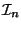of intervals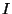of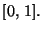.